Here we are providing Class 11 chemistry Important Extra Questions and Answers Chapter 1 Some Basic Concepts of Chemistry. Chemistry Class 11 Important Questions are the best resource for students which helps in Class 11 board exams.

## Class 11 Chemistry Chapter 1 Important Extra Questions Some Basic Concepts of Chemistry

### Some Basic Concepts of Chemistry Important Extra Questions Very Short Answer Type

Question 1.
What is the number of significant figures in 1050 × 104?
Four.

Question 2.
What is the SI unit of length?
Metre.

Question 3.
What is the SI unit of density?
kg m-3.

Question 4.
What is the law called which deals with the ratios of volumes of the gaseous reactants and products?
Gay Lussac’s law of combining volumes.

Question 5.
What is one a.m.u. or one u?
1 a.m.u. or 1 u = $$\frac{1}{12}$$th of the mass of an atom C-12.

Question 6.
Which isotope of carbon is used for getting relative atomic masses?
Carbon-12 (C-12).

Question 7.
Write down the empirical formula of benzene.
CH.

Question 8.
The mass of human DNA molecules is 1 FG. What is its mass in kg?
10-18 kg

Question 9.
Vanadium metal is added to steel to impart strength. The density of vanadium is 5.96 g/cm3. Express this in the SI unit.
5960 kg/m3.

Question 10.
What physical quantities are represented by the following units and what are their most common names?
(i) kg ms2
Force (Newton)

(ii) kg m2s2.
Work (joule)

Question 11.
If in the reaction HgO(s) → Hg(l) + $$\frac{1}{2}$$O2(g) 100.0 g of HgO on heating in a closed tube gives 92.6 g of Hg, what is the weight of oxygen formed?
Weight of oxygen formed = 100 – 92.6 = 7.4 g.

Question 12.
Name three compounds formed by dinitrogen and dioxygen.
1. N2O (Nitrous Oxide)
2. NO (Nitric Oxide)
3. N2O5 (Nitrogen pentoxide).

Question 13.
If 700 mL of H2 at STP contains x molecules of it, how many molecules of O2 are present in 700 mL of it at the same temperature and pressure?
x molecules.

Question 14.
nitrogen combines with dihydrogen according to the reaction.
N2(g) + 3H2(g) ⇌ 2NH3(g)
What is the ratio in their volumes under similar conditions of temperature and pressure?
The ratio in their volumes is 1: 3: 2.

Question 15.
What is the relationship between molecular weight and vapor density of a gas?
Molecular weight = 2 × Vapour density.,

Question 16.
What is the mass in gms of 11.2 L of N2 at STP?
22.4 L of N2 at STP weighs = 28.0 g.
11.2 L of N2 at STP weighs = $$\frac{28}{22.4}$$ × 11.2 = 14.0 gm.

Question 17.
What is the mass of one molecule of sodium chloride?
6.023 × 1023 molecules of NaCl weighs = 58.5 g

I molecule of NaCI weighs = $$\frac{58.5}{6.023 \times 10^{23}}$$ = 9.71 × 10-23 g.

Question 18.
How many total electrons are present in 1.4 g of nitrogen gas?
1.4 g of N2 = $$\frac{14}{28}$$ mol = 0.05 and 0.05 × 6.02 × 1023 molecules
= 3.01 × 1021 molecules
= 3.01 × 1021 × 14 electrons [ ∵ 1 Molecules of 2 = 14 ē]
= 4.214 × 1022 electrons.

Question 19.
Why atomic masses are the average values?
Most of the elements exist in different isotopes. Hence their average values are taken.

Question 20.
What is the percentage composition of Ca in CaCO3?
% of Ca = $$\frac{40 \times 100}{40+12+3 \times 16}$$ = 40.0%

Question 21.
What is the mass of 2 moles of CO2?
1 mole of CO2 = 1 × 12 + 2 × 16 = 44 g.
2 mole of CO2 = 2 × 44.0 = 88.0 g.

Question 22.
Express the number 45000 in exponential notation to show
(i) two significant figures
4.5 × 104

(ii) four significant
4.500 × 104.

Question 23.
How many moles of sulphuric acid are present in 1 dm3 of 0.5 M solution?
0.5 mole.

Question 24.
Give one example each of a molecule in which empirical formula and molecular formula are
(i) different
(ii) same.

 Compound Empirical formula Molecular formula (i) Hydrogen peroxide HO H2O2 (ii) Copper sulfate CuSO4 CuSO4.

Question 25.
The radius of an atom is 10-10 m. What will be its value in micro metre?
10-10 m = $$\frac{10^{-10}}{10^{-6}}$$ = 10-4 micrometer.

Question 26.
Define the limiting reagent.
The reacting substance gets used up first in the reactions.

Question 27.
What is meant by 1 gram atom of iron?
1 g atom of iron means the atomic mass of iron expressed in g i.e., 56 g.

Question 28.
What is the number of oxygen atoms in one mole of CuSO4.5H2O?
It is moles of oxygen atoms = 9 × 6.02 × 1023 = 5.418 × 1024 atoms.

Question 29.
What is the SI unit of velocity?
ms-1.

Question 30.
A worker reads the resistance of the wire as 20.01 ohm. What may be the actual range of resistance?
20.01 ± 0.01 ohm, i.e., 20.00 to 20.02 ohm.

Question 31.
What is AZT? To which use is it put?
Azidothymidine used for AIDS victims.

Question 32.
Write down the empirical formula of acetic acid.
CH2O.

Question 33.
What is the advantage of using Molality over Molarity?
Molality does not depend upon temperature.

Question 34.
Balance the equation
C3Hg(g) + O2(g) → CO2(g) + H2O(1)
C3H8(g) + 5 O2(g) → 3 CO2(g) + 4 H2O(l)

Question 35.
Balance the equation
Fe(s) + O2(g) → Fe2O3(s)
4 Fe(s) + 3 O2(g) → 2 Fe2O3(s)

Question 36.
Balance the equation
P4(s) + O2(g) → P4O10(s)
P4(s) + 5 O2(g) → P4O10(s)

Question 37.
What is the SI scale of temperature?
Kelvin (K).

Question 38.
What is the relation between Empirical formula and molecular formula?
Molecular formula = n × Empirical formula
where n = $$\frac{\text { Molar mass }}{\text { empirical formula mass }}$$

Question 39.
What is the mass of NH3 in 0.5 mol of it?
0.5 mol of NH3 weights 8.5 g.

Question 40.
What is the mass of 3.01 × 1022 g of SO2?
3.2 g.

Question 41.
How many atoms of silver are present in a 0.5 g atom of silver? At the mass of silver = 108.
3.01 × 1023 atoms.

Question 42.
Which of the two is heavier – 2 g of iron or 2 g atom of iron.
2 g atom of iron.

Question 43.
How many molecules of CO2 are present in 1.12 L of it at STP?
3.011 × 1022 molecules.

Question 44.
What is the weight of H2SO4 in 0.01 M solution of it?
0.98 g.

Question 45.
What volume of CO2 at STP can be obtained on the decomposition of 100.0 g of CaCO3?
22.4 L.

Question 46.
How many molecules of cane sugar (C12H22O11) are present in 34.20 grams of it.
6.022 × 1022 molecules.

Question 47.
How many molecules of water are present in a drop of it having a mass of 0.05 g?
1.673 × 1021 molecules.

Question 48.
Calculate the mass of 0.1 moles of KNO3.
[At. wt. of K = 39, N = 14, O = 16]
1 Mole of KNO-, = 1 × 39 + 1 × 14 + 3 × 16 = 101 g
∴ 0.1 mole of it = 101 × 0.1 = 10.1 g.

Question 49.
What is the molarity of a solution of oxalic acid containing 0.63 g of it in 250 cm3 of the solution?
Molarity = 0.02 M.

Question 50.
A solution of NaCl has been prepared by dissolving 5.85 g of it 1 L of water. What is its molality?
0.1 m.

### Some Basic Concepts of Chemistry Important Extra Questions Short Answer Type

Question 1.
Define Mole. What is its numerical value?
A mole is the amount of a substance that contains as many entities (atoms, molecules, or other particles) as there are atoms in exactly 0.012 kg or 12 g of the carbon-12 isotope.

Its numerical value is 6.023 × 1023.

Question 2.
Define molarity. Is it affected by a change in temperature?
The molarity of a solution is defined as the number of moles of the solute present per liter of the solution. It is represented by the symbol M. Its value changes with the change in temperature.

Question 3.
What do you mean by Precision and accuracy?
Precision and accuracy: The term precision refers to the closeness of the set of values obtained from identical measurements of a quantity.

Accuracy refers to the closeness of a single measurement to its true value.

Question 4.
Distinguish between fundamental and the derived units.
Fundamental units: Fundamental units are those units by which other physical units can be derived. These are mass (M), Length (L), time (T), temperature (°).

Derived units: The units which are obtained by the combination of the fundamental units are called derived units.

Question 5.
Define molality and write its temperature dependence.
Molality is defined as the number of g moles of the solute dissolved per kilogram of the solvent.
Molality (m) = $$\frac{\text { Mole of solute }}{\text { Mass of the solvent in } \mathrm{kg}}$$

The molality of the solution does not depend upon the temperature.

Question 6.
Distinguish between an atom and a molecule.
Atom: An atom is the smallest particle of an element that takes part in a chemical reaction. It may or may not be capable of independent existence.

Molecule: It is the smallest particle of a substance (element or compound) that is capable of independent existence

Question 7.
Derive the SI unit of Joule (J) in terms of fundamental units.
Joule is the SI unit of work or energy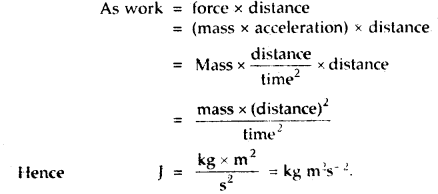Question 8.
Define the SI unit of energy.
Energy = Force × distance through which point of application of force moves.
S.l. unit of energy = SI unit of force × SI unit of distance.
= 1 Newton × 1 metre
= 1 Nm

But 1 N = 1 kg ms-2
∴ SI unit of energy = 1 kg ms-2 × 1 m = 1 kg m2s-2

The unit of energy is joule (J)
Thus 1 Joule (J) = 1 kg m2 s-2.

Question 9.
One volume of a gaseous compound requires 2 volumes of O2 for combustion and gives 2 volumes of CO2 and 1 volume of N22. Determine the molecular formula of the compound.
X + 2O2 → 2 CO2 + N2 (According to problem)
Since the product has 2 atoms of C (in 2 CO2) and 2 atoms of N (in N2) these must have come from X. Hence X is C2N2.

Question 10.
What is a chemical equation? What are its essential features?
The shorthand representation of a chemical change in terms of symbols and formulae is called a chemical equation.

Essential features:

• It should represent a true chemical change.
• It should be balanced.
• It should be molecular.

Question 11.
Write down the significance of a chemical equation.
Significance of a chemical equation:

1. It tells about the reactants taking part in the chemical reaction and products formed as a result of chemical change.
2. It tells about the relative number of atoms or- molecules of reactants and products.
3. It tells about the relative weights of reactants and products

Question 12.
How is mole related to
(a) number of atoms/molecules
A mole contains 6.022 × 1023 atoms/molecules.

(b) mass of the substance?
A mole of a substance denotes the molecular mass of that substance.

Question 13.
How is mole related to the volume of a gas?
A mole of a gas occupies 22.4 L of the gas measured at STP, i. e., 0° C and 1 atmospheric pressure.

Question 14.
Why atomic masses are the average values?
Most of the elements exist in different isotopic forms. Chlorine has 2 isotopes with mass numbers 35 and 37 existing in the ratio of 3: 1. Hence the average value is taken.

Question 15.
How many molecules approximately do you expect to be present in a small crystal of sugar which weighs 10 mg?
10 mg sugar (C12H22O11) = 0.01 g = $$\frac{0.01}{342}$$ mol
= 2.92 × 10-5 mole
= 2.92 × 10-5 × 6.02 × 1023 molecules
= 1.76 × 1019 molecules.

Question 16.
Two containers of equal capacity A1 and A2 contain 10 g of oxygen (O2) and ozone (O3) respectively. Which of the two will have greater no. of O-atoms and which will give greater no. of molecules?
10 g of O2 = $$\frac{10}{32}$$ mol = $$\frac{10}{32}$$ × 6.02 × 1023 molecules
= 1.88 × 1023 molecules
= 3.76 × 1023 atoms.

10 g of O2 = $$\frac{10}{48}$$ mole = $$\frac{10}{48}$$ × 6.02 × 1023 molecules
= 1.254 × 1023 molecules
= 3.76 × 1023 atoms

Thus both A1 and A2 contain the same no. of atoms, but A1 contains more numbers of molecules.

Question 17.
What do you mean by the term ‘Formality’? To what type of compounds it is applied?
Ionic compounds like Na+Cl are not molecular. To express the concentration of their solution the term formality is used in place of molarity. Formality is the number of formula weights present in one liter of a solution.

Question 18.
What is meant by a ‘standard solution’?
A standard solution is one whose molarity or normality is known. For example 0.1 M NaOH.

Question 19.
Assuming the density of water to be 1 g/cm3, calculate the volume occupied by one molecule of water.
1 Mole of H2O = 18 g = 18 cm3[∵ density of H2O = 1 g/cm3]
= 6.022 × 1023 molecules of H2O
1 Molecule will have a volume
= $$\frac{18}{6.022 \times 10^{23}}$$ cm- = 2.989 × 10-23 cm3.

Question 20.
Define Avogadro’s number. What is its equal to?
Avogadro’s number may be defined as the number or number of molecules present in one gram molecule of the substance.
Avogadro’s No. = 6.022 × 1023.

### Some Basic Concepts of Chemistry Important Extra Questions Long Answer Type

Question 1.
State the law of Multiple Proportions. Explain with two examples.
The Law of Multiple Proportions states:
“When two elements combine to form two or more than two chemical compounds than the weights of one of elements which combine with a fixed weight of the other, bear a simple ratio to one another.

Examples:
1. Compound of Carbon and Oxygen: C and O combine to form two compounds CO and CO2.
In CO2 12 parts of wt. of C combined with 16 parts by wt. O.
In CO2 12 parts of wt. of C combined with 32 parts by wt. of O.
If the weight of C is fixed at 12 parts by wt. then the ratio in the weights of oxygen which combine with the fixed wt. of C (= 12) is 16: 32 or 1: 2.
Thus the weight of oxygen bears a simple ratio of 1: 2 to each other.

2. Compounds of Sulphate (S) and Oxygen (O):
S forms two oxides with O, viz., SO2 and SO3
In SO2, 32 parts of wt. of S combine with 32 parts by wt. of O.
In SO3, 32 parts of wt. of S combine with 48 parts by wt. of O.
If the wt. of S is fixed at 32 parts, then’ the ratio in the weights of oxygen which combine with the fixed wt. of S is 32: 48 or 2: 3.

Thus the weights of oxygen bear a simple ratio of 2: 3 to each other.

Question 2.
State the law of Constant Composition. Illustrate with two examples.
Law of Constant Composition of Definite Proportions states: “A chemical compound is always found, to be made up of the same elements combined together in the same fixed proportion by weight”.

Examples:

1. CO2 may be prepared in the laboratory as follows: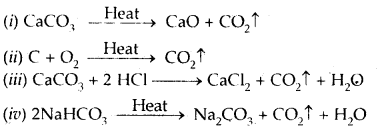In all the above examples, CO2 is made up of the same elements i. e., Carbon (C) and Oxygen (O) combined together in the same fixed proportion by weight of 12: 32 or 3: 8 by weight.

Question 3.
Define empirical formula and molecular formula. How will you establish a relationship between the two? Give examples.
The empirical formula of a compound expresses the simplest whole-number ratio of the atoms of the various elements present in one molecule of the compound.

For example, the empirical formula of benzene is CH and that of glucose is CH2O. This suggests that in the molecule of benzene one atom of Carbon (C) is present for every atom of Hydrogen (H). Similarly in the molecule of glucose (CH2O), for every one atom of C, there are two atoms of H and one atom of O present in its molecule. Thus, the empirical formula of a compound represents only the atomic ratio of various elements present in its molecule.

The molecular formula of a compound represents the true formula of its molecule. It expresses the actual number of atoms of various elements present in one molecule of a compound. For example, the molecular formula of benzene is C6H6 and that of glucose is C6H12O6. This suggests that in one molecule of benzene, six atoms of C and 6 atoms of H are present. Similarly, one molecule of glucose (C6H12O6) actually contains 6 atoms of C, 12 atoms of H, and 6 atoms of O.

Relation between the empirical and molecular formula
Molecular formula = n × Empirical formula where n is an integer such as 1, 2, 3…
When n = 1; Molecular formula = Empirical formula
When n = 2; Molecular formula = 2 × Empirical formula.
The value of n can be obtained from the relation.
n = $$\frac{\text { Molecular mass }}{\text { Empirical formula mass }}$$

The molecular mass of a volatile substance can be determined by Victor Meyer’s method or by employing the relation.
Molecular mass = 2 × vapour density .

Empirical formula mass can however be obtained from its empirical formula simply by adding the atomic masses of the various atoms present in it.

Thus the empirical formula mass of glucose CH20
= 1 × 12 + 2 × 1 + 1 × 16 = 30.0 u.

### Some Basic Concepts of Chemistry Important Extra Questions Numerical Problems

Question 1.
In the commercial manufacture of nitric acid, how many moles of NO2 produce 7.33 mol HN03 in the reaction
3 NO2(g) + H2O(1) → 2HNO3(aq) + NO(g).
2 mols of HNO3 are produced by 3 mols of NO2
7.33 mol HNO3 are produced by $$\frac{3 \times 7.33}{2}$$ mol of NO2
= 10.995 mols.

Question 2.
A sample of NaNO3 weighing 0.83 g is placed in a 50,0 mL volumetric flask. The flask is then filled with water upon the etched mark. What is the molarity of the solution?
Molar mass of NaNO3 = 23 + 14 + 3 × 16 = 85 g mol-1

Molarity = $$\frac{\text { Number of moles of solute }}{\text { Volume of solution in } L}$$
= $$\frac{0.83 \times 1000}{85 \times 50}$$
= 0.196 M.

Question 3.
Potassium bromide’ KBr contains 32.9% by mass of potassium. If 6.40 g of bromine reacts with 3.60 g of potassium, calculate the number of moles of potassium that combine with bromine to form KBr.
Mass percentage of K = 32.9
∴ Mass percentage of Br = 100 – 32.9 = 67.1
Now 67.1 g of bromine combines with 32.9 g of potassium

6.40 g of bromine combines with = $$\frac{32.9}{67.1}$$ × 6.40 = 3.14 g of K
Potassium (K) which remains unreacted = 3.60 – 3.14 = 0.46 g

Thus, Br2 is the limiting reagent.
Mass of K = $$\frac{\text { Mass of } \mathrm{K}}{\text { Atomic mass of } \mathrm{K}}=\frac{3.14 \mathrm{~g}}{39 \mathrm{~g} \mathrm{~mol}^{-1}}$$
= 0.0802 mol.

Question 4.
Calculate the molarity of water in a sample of pure water.
1 L of pure water = 1000 cm3
= 1000 g (assuming density = 1 g cm-3)

No. of moles of H2O in pure water = $$\frac{1000}{18.0}$$ = 55.55 L-1

Question 5.
How many molecules are there in 10.0 liters of a gas at a pressure of 75 cm at 27°C?
PV = nRT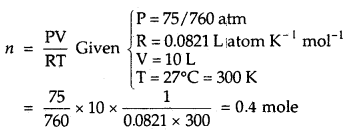∴ Number of molecules present in 0.4 mol
= 6.023 × 1023 × 0.4 = 2.409 × 1023 molecules.

Question 6.
Two acids H2SO4 and H3PO4 are neutralized separately by the same amount of an alkali when sulfate and dihydrogen orthophosphate are formed respectively. Find the ratio of the masses of H2SO4 and H3PO4 (P = 31).
1 g equivalent of alkali (NaOH) will neutralize 1 g Eqn. of H2SO4 and 1 g eq. of H3PO4. For the given neutralization reaction.
H2SO4 + 2 NaOH → Na2SO4 + 2 H2O
H3PO4 + NaOH = Na H2PO4 + H2O

Eq. wt. of H2SO4 = $$\frac{\text { Mol. wt. }}{2}=\frac{98}{2}$$ = 49;
Eq wt. of H3PO4 .
$$\frac{\text { Mol. wt. }}{1}$$ = 98

Hence the ratio of masses of H2SO4 and HPO4 = 49: 98 = 1: 2.

Question 7.
What weight of iodine is liberated from a solution, of potassium iodide when 1 liter of Cl2 gas at 10° C and 750 mm pressure is passed through it?
The chemical equation is
2KI + Cl2 → 2KCl + I2
22.4 L   2 × 127
at STP = 254 g

(V1) volume of Cl2 gas=1 L
V2 = vol. of gas at
STP =?

(P1) Pressure = $$\frac{750}{760}$$atm
P2 = 1 atm

(T1) Temperature = 10°C + 273 = 283 K
T2 = 273 K

∴ V2 = $$\frac{P_{1} V_{1}}{T_{1}^{-}} \times \frac{T_{2}}{P_{2}}=\frac{750 \times 1 \times 273}{760 \times 283 \times 1}$$
= 0.952 L

∴ Vol of Cl2 g passed at STP = 0.952 L
Now 22.4 L of Cl2 produces at STP = 254 g of I2

0.952 L of Cl2 at STP produces = $$\frac{254}{22.4}$$ × 0.952 g of I2
= 10.78 g of I2.

Question 8.
A crystalline salt on being rendered anhydrous loses 45.6% of its weight. The percentage composition of the anhydrous salt is Aluminium = 10.50%, Potassium = 15.1% Sulphur = 24.96%, Oxygen = 49.92%. Find the simplest formula of the anhydrous and crystalline salt.
Step 1: To calculate the empirical formula of the anhydrous salt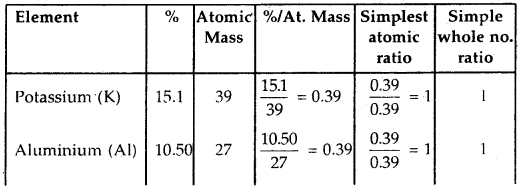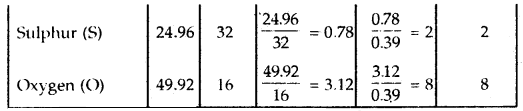The empirical formula of the anhydrous salt is KAlS2O8

Step 2: Empirical formula mass of anhydrosis salt
= 39 + 27 + 2 × 32 + 8 × 16 = 258 u

Step 3: To calculate the empirical formula mass of the hydrated salt.
Loss of water due to dehydration = 45.6%

∴ The empirical formula mass of anhydrous salt (assuming empirical formula mass of the hydrated sample to be 100) = 100 – 45.6 = 54.4 u

When empirical formula mass.of anhydrous salt is 258 u, that of 100 hydrated is = $$\frac{100}{54.4}$$ × 258 = 473.3 u

Step 4: To calculate the no. of water molecules
Total loss in wt. due to dehydration = 473.3 – 258 = 215.3
No. of water molecules = $$\frac{215.3}{18}$$ = 11.96 ≈ 12

Step 5: Empirical formula of the hydrated salt = KAlS2O8.12H2O

Question 9.
An organic compound containing C, H, and O gave the following percentage composition: C = 40.687%.
H = 5.085% O = 54.228%
The vapor density of the compound is 59. Calculate the molecular formula of the compound.
Calculation of the empirical formula of the compound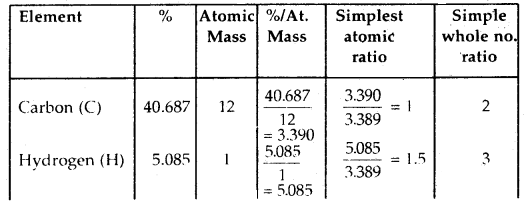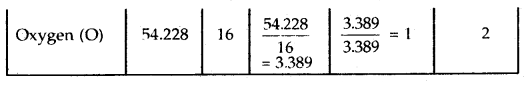∴ The empirical formula of the compound is C2H3O2
Molecular massof compound = 2 × V.D. = 2 × 59 = 118
n = $$\frac{\text { Molecular Mass }}{\text { Empirical formula mass }}$$
= $$\frac{118}{2 \times 12+3 \times 1+2 \times 16}=\frac{118}{59}$$ = 2

∴ Molecular formula of the compound
= n × empirical formula = 2 × C2H3O2
= C4H6O4.

Question 10.
Calculate the number of molecules present in 350 cm3 of NH3 gas at 273 K and 2-atmosphere pressure.
Calculate the volume of the gas at STP.
V1 = 350 cm3
V2 = ?
T1 = 273 K
T2 = 273 K
P1 = 2 atm.
P2 = 1 atm

V2 = $$\frac{P_{1} V_{1}}{T_{1}} \times \frac{T_{2}}{P_{2}}=\frac{2 \times 350 \times 273}{273 \times 1}$$ = 700 cm3

22,400 cm3 of NH3 at STP contains
= 6.023 × 1023 molecules of NH3

700 cm3 of NH3 at STP will contain
= 1.882 × 1022 molecules.

Question 11.
Calculate the percentage of water of crystallization in the sample of blue vitriol (CuS04.5H20).
Mol. wt. of CuSO4.5H2O) = 63.5 + 32 + 4 × 16 + 5 × 18 = 249.5
No. of parts bv wt. of H2O = 5 × 18 = 90
∴ % of H2O = $$\frac{90}{249.5}$$ × 100 = 36.07

Question 12.
Calculate the weight of iron which will be converted into its oxide (Fe3O4) by the action of 18 g of steam on it.
The chemical equation representing the reaction is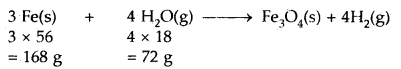Now 72 g of steam [H2O(g)] react with 168 g of Iron

∴ 18 g of steam will’reacts with = $$\frac{168}{72}$$ × 18 = 42.0 g of iron
Thus, the weight of iron required = 42.0 g

Question 13.
To account for the atomic mass of nitrogen as 14.0067, what should be the ratio of 15N and 14N atoms in natural nitrogen?
[At. mass of 14N = 14.00307 u and 15N = 15.001 u]
Let the % of 14N = x
% of 15N = 100 – x
According to the definition of average atomic mass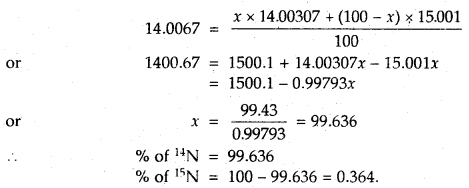Question 14.
2.16 g of copper metal, when treated with nitric acid followed by ignition of the nitrate, gave 2.70 g of copper oxide. In another experiment, 1.15 g of copper oxide upon reduction with hydrogen gave 0.92 g of copper. Show that the above data illustrates the law of definite proportions.
% of Cu in copper oxide in 1st case = $$\frac{2.16 \times 100}{2.70}$$ = 80
% of oxygen = 20%
% of Cu in copper oxide in 2nd case = $$\frac{0.92 \times 100}{1.15}$$ = 80
% of oxygen = 20%

Thus, the percentage of copper in copper oxide from both experiments is the same. Hence the above data illustrates the law of definite proportions.

Question 15.
The mass of precious stones is expressed in terms of ‘carat’. Given that 1 carat = 3.168 grains and 1 gram = 15.4 grains, calculate the total mass of a ring in grams and kilograms which contains 0.500 carat diamond and 7.00 gram gold.
The unit conversion factors to be used will beQuestion 16.
“The star of India” sapphire weighs 563 carats If one carat is equal to 200 mg, what is the weight of the gemstone in grams?
The weight of the gemstone in grams will be
= 563 carats × $$\frac{200 \mathrm{mg}}{1 \text { carat }}=\frac{1 \mathrm{~g}}{100 \mathrm{mg}}$$
= 112.6 g

Question 17.
When 4.2 g of NaHCO3 is added to a solution of acetic acid (CH3COOH) weighing 10.0 g, it is observed that 2.2 g of CO2 is released into the atmosphere. The residue left behind is found to weigh 12.0 g. Show that these observations are in agreement with the law of conservation of mass.Total mass of the reactants = 10.0 + 4.2 = 14.2 g
Total mass of the products = 12.0 + 2.2 = 14.2 g

Thus, during the above chemical reactions, the total mass of the reactants is equal to the total mass of the products, i.e., the matter is neither gained nor lost.

This is in keeping with the law of conservation of mass.

Question 18.
Calculate the volume of 0.05 M KMnO4 solution required to oxidize completely 2.70 g of oxalic acid in acidic solution.
The equation representing the chemical change is
2KMnO4 + 3 H2SO4 → K2SO4 + 2 MnSO4 + 3 H2O = 5
H2C2O4 + [O] → 2 CO2 + H2O × 5

Step I. To calculate the no. of moles of KMn04 required to completely oxidize 2.70 g of’H2C2O4 in acidic medium:
Molar mass of H2C2O4 = 2 × 1 +2 × 12 + 4 × 16
= 90.0 g mol-1

∴ No. of moles of H2C2O4 contained in 2.70 g of it = $$\frac{2.70}{90.0}$$ = 0.03

5 Moles of H2C2O4 are oxidized by 2 moles of KMnO4
∴ 0.03 moles of it oxidized by = $$\frac{2}{5}$$ × 0.03
= 0.012 mole of KMnO4

Step II. To calculate the volume of 0.05 M KMnO4 solution
Now 0.05 mole of KMnO4 are contained in 1000 cm3 of solution
∴ 0.012 mole of KMnO4 will be contained in
$$\frac{1000}{0.05}$$ × 0.012 = 240 cm3 of solution

Thus, the required volume of 0.05 M KMnO4 solution = 240 cm3.

Question 19.
4 g carbon was heated with 8 g of sulfur. How much carbon disulfide (CS2) will be formed when the reaction is complete? What will be its percentage purity?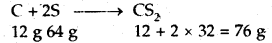Obviously sulphur will be limiting reactant.

8 g of sulphur will produce CS2 = $$\frac{76}{64}$$ × 8 = 9.5 g

Amount of carbon reacted = $$\frac{12}{64}$$ × 8 = 1.5g

Amount of carbon left = 4 – 1.5 = 2.5 g
Total weight of the products = 9.5 + 2.5 = 12 g

% purity of CS2 in the product = $$\frac{9.5}{12}$$ × 100 = 79.2.

Question 20.
A gas mixture of 3.0 liters of propane and butane on complete combustion at 25°C produced 10 liters of CO2. Find out the composition of the gas mixture.
C4H10 + 6 $$\frac{1}{2}$$O2 → 4 CO2 + 5 H2O